By Martin McBride, 2022-11-20
Tags: quadrilateral square rectangle parallelogram rhombus trapezium isosceles trapezium kite
Categories: gcse geometryWe previously looked at the different types of quadrilaterals. In this article, we will look at how these different shapes relate to each other, in the form of a family tree.

As we go down the tree, each new shape is a special type of its parent (or parents). It inherits its parent's characteristics while also adding new ones of its own.

Here is the family tree: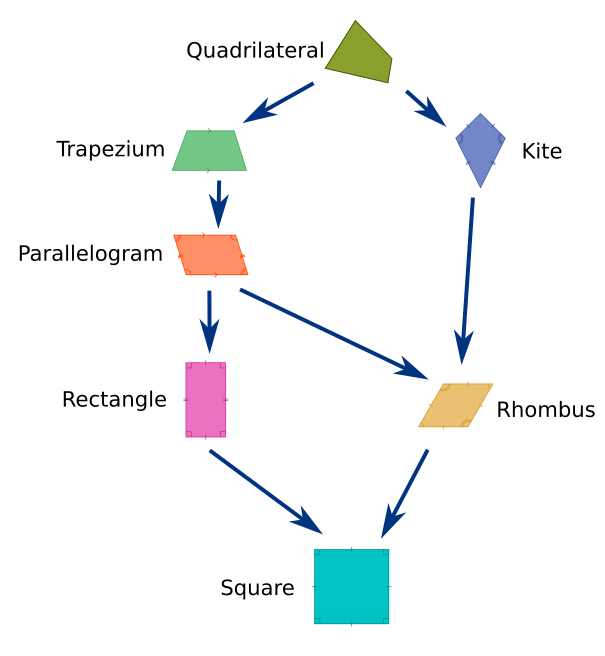A quadrilateral is any shape with four sides. It is the most general type of four-sided shape.

## Trapezium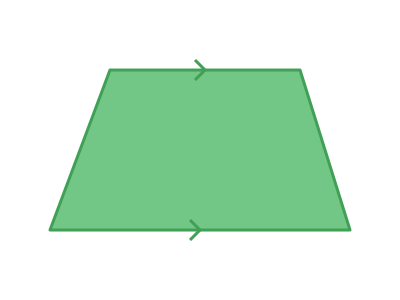A trapezium is a special type of quadrilateral where one pair of opposite sides are parallel.

## Parallelogram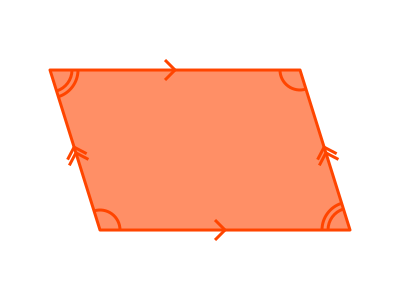A parallelogram is a special type of trapezium where both pairs of opposite sides are parallel.

Each pair of opposite sides have the same length.

## Kite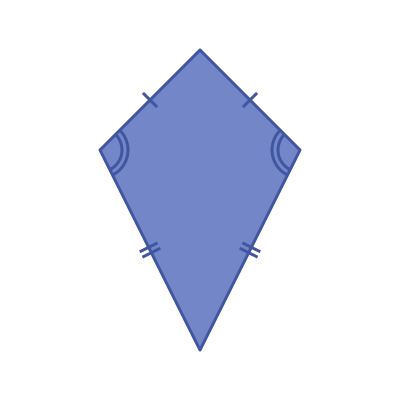There are two equivalent definitions of a kite.

1. A kite is a special type of quadrilateral that has two pairs of equal adjacent sides.
2. A kite is a special type of quadrilateral in which one of its diagonals is a line of symmetry.

## Rhombus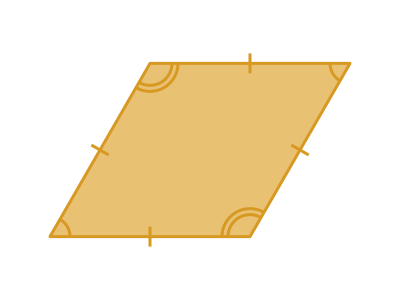A rhombus is a special type of parallelogram and also a special type of kite.

• A parallelogram has two pairs of opposite sides that have equal lengths.
• A kite has two pairs of adjacent sides that have equal lengths.

In a rhombus, all four sides are equal. This allows it to satisfy both conditions at the same time.

## Rectangle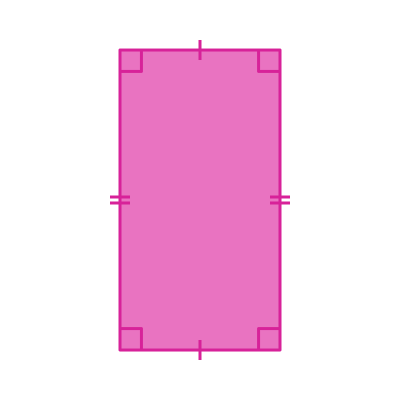A rectangle is a special type of parallelogram that has four 90-degree angles. Like a parallelogram, the two pairs of opposite sides have equal lengths.

## Square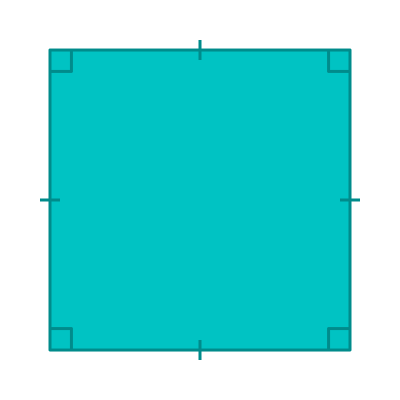A square is a special type of rectangle that has all four sides the same length.

It is also a special type of rhombus that has four 90-degree angles.

A square inherits its four 90-degree angles from the rectangle and its four sides of equal length from the rhombus.

All squares are the same shape. This means a square is the most specialised quadrilateral.